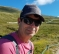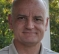## DTFT optimization questionStarted by 3 years ago16 replieslatest reply 3 years ago184 views

Hello Everyone,

I am using cuda on a Jetson Xavier AGX to do some signal processing. I am processing a time series which is 16 bit samples taken at 1 GHz. Each time I receive 8192 samples I am given 10 frequency locations. I break the 8192 samples into 4 sections and calculate 10 DTFTs for each section. The frequency locations I am given are bin centers for a 8192 FFT (So they are integer multiples of 122 kHz). So we have 4 sets of 10 coefficients for each 8192 samples, all on bin centers for a 8us FFT. I have this working using cuBLAS as the core of my DTFT calculation.

The physicist have now decided they need 4 sets of 70 coefficients for each 8192 samples. I cannot make this work on the AGX using my old DTFT method. I proposed calculating 2048 FFTs and extracting the coefficients but of course these are not on 122kHz bin centers. I could zero pad a 8192 array and put in the 2048 section and do the FFT but this requires me to do 4 8192 FFTs every 2.048 us. (and  all my other signal processing). Unfortunately, the AGX can’t keep up with this.

Does anyone have an idea on a more efficient way to calculate the coefficients? I am told which frequencies / bins I need when I receive the time samples. The 10 frequencies where I need to calculate change for each 8 us sample block. Basically, I need to do 280 DTFT’s (or something equivalent) every 8us. Calculation latency can be pretty long 100 ms would be fine.

[ - ]If I understood correctly you are given 10 frequencies and want to calculate the (complex?) FT of a signal of length 8192. I am not sure what "break the signal into 4 sections" means. Do you mean decimate into 4 subsequences? Partition into 4 subsequences?

In any case, for calculating only 10 frequencies our of 8192 do NOT use the standard FFT. The radix-2 DIT or DIF is only worthwhile when you need over 25% of the coefficients. For only a few bins even the straightforward DFT convolution is faster. For a somewhat larger number there are various special algorithms.

The best known way to find only a few bins is Goertzel's algorithm (which requires N multiplies and 2N adds per frequency).

Y(J)S

[ - ]I am partitioning the sequence into 4 sub-sequences 0-2047, 2048-4095, 4096-6143,6144-8191.  I have real input and compute complex coefficients.

I will look at implementing Goertzel's algorithm. I  found a pseudocode example and I think I can implement a real-valued input version efficiently for my GPU.

Thanks again for the help.

[ - ]Bear in mind that the Goertzel algorithm may only require N multiplies and 2N adds per frequency, but -- being an IIR filter -- it will, in general, require a wider data path, and it cannot be easily pipelined.  If you have to increase the datapath width those "thrifty" operations may take more time than more (and pipelined) operations on narrower datapaths.

It's a good trick to have in your bag-o-tricks, but it's not a be-all end-all solution.

[ - ]Goertzel isn't an IIR filter. It is a recusive method of evaluating a polynomial in W_N. Polynomial evaluation is FIR (the coefficients - the powers of W_N are convolved with the inputs); but Horner's rule changes that FIR evaluation into a recursive form.

But I totally agree that Goertzel isn't the only way. As I said it may even be good enough to simply compute the straightforward DFT at the desired frequencies (using a table or using Horner's rule). Goertzel merely saves some computation by changing the complex Horner evaluation into a real recursion.

[ - ]"Goertzel isn't an IIR filter. It is a recursive method of evaluating a polynomial in W_N."

An IIR filter is a recursive method of evaluating a polynomial in W_N.  Except at the endpoints, a Goertzel filter uses exactly the same difference equation as an IIR bandpass filter with zero damping factor.

So it looks like a duck, it quacks like a duck, it has feathers the same as a duck, it's DNA has the same sequence as duck DNA -- but you say it's not a duck?

[ - ]Again, I agree Tim.

I view Goertzel as a "sympathetic" driven oscillator.
The poles are on the unit circle (zero damping as you said) as they would be if you chose to implement an oscillator using a biquad.

The difference is that there is an input to the oscillator that forces its response to be in phase with, and at the level of, the input signal.

If a pure oscillator is needed, and a specific starting phase and amplitude is required, then the starting values of the 2 internal states must be calculated and used to initialize the process.

[ - ]Oh my god!  I just realized that a Goertzel is pretty darned close to a super-regenerative receiver, only with the poles on the stability boundary instead of outside of it.

We keep thinking we're inventing new stuff -- but no, we're just mining stuff that's been done in the past.

[ - ]MA filters can be formally written in ARMA format, but that doesn't make them full ARMA.

I hope that you agree that the simple causal 3-point average with gain 3

y_n =  x_{n-2} + x_{n-1} + x_n

is a FIR filter.

It is simple to rewrite this

y_n = y_{n-1} + x_n - x_{n-3}

which contains the previous output.

So, is this IIR?

The Goertzel filter is precisely the MA convolution of the DFT, just rearranged to save computation.

[ - ]Thanks Tim,
I totally agree, and you still have to run the correct number of samples through it to get to the right result.

Just thought it might be an option for a small subset of the total BW of the signal, and is very flexible.

David

[ - ]I just looked up the details of the computing platform described (Jetson Xavier AGX) in the original problem statement, and it appears to have several floating point options, so I think the feedback issue in biquads might survive the wider data-path issue you raise.

[ - ]I have absolutely nothing against the Goertzel itself.  I do think that people tend to over-apply it; if you're thinking of using it you should do so in the context of it and its alternatives.  After analyzing what's best, you shouldn't hesitate to use something else -- just as you shouldn't hesitate to use the Goertzel if it wins out in the analysis.

[ - ]Look into the Sliding DFT algorithm.   It is a recursive sliding-window computation that greatly reduces the computational load for sparse outputs (like what you are describing).

Alternatively, you could just compute the required output bins for the selected windows with the ordinary DFT algorithm.   The advantage is that you only need to compute the desired output bins and skip any computations for other bins.

Since the difference between the sliding-DFT and the ordinary DFT in this regard is that the sliding DFT provides a valid output for that coefficient after every new input.  i.e., for each new input, the output coefficient is equivalent to the same DFT coefficient for the previous N samples.

When the output is highly sparse, i.e., you only compute a fraction of the full N DFT outputs, the savings can be dramatic compared to the usual FFT algorithms where the savings is made by sharing computations across all output bins.

[ - ]A Goertzel filter is effectively a 1 bin FT.
Have you looked into it for what you need?
It is effectively implemented using a biquad section for each desired frequency.
All you need is a table of coefficients into which you can index for the specific frequencies of interest, and you can pick out any you want at random.

"For covering a full spectrum, the Goertzel algorithm has a higher order of complexity than fast Fourier transform (FFT) algorithms, but for computing a small number of selected frequency components, it is more numerically efficient."

[ - ]You say you need four sets of 70 coefficients, but you don't say what those are -- do you mean four sets of DTFT results at 70 different frequencies?

[ - ]I think I can describe the desired FT coefficients more precisely. I am given the 8192 sample time series (sampled at 1 GHz) and 10 frequencies (These frequencies are on bin centers if you were to do a FFT of the 8192 sample time series)

I am partitioning the time series into 4 sub-sequences 0-2047, 2048-4095, 4096-6143,6144-8191.

I need the FT coefficients for each of the sub-sequences, phase and magnitude, for the frequencies I am given and three “bins” on either side. Each “bin” is 122.0703125 kHz. So 7 coefficients per frequency for each sub-sequence. 70 frequencies x 4 sub-sequencies = 280 total coefficients

If you think about the radix-2 FFT for the entire 8192 time series these sub-sequence coefficients are intermediate results that would be part of the calculation. If you take 4 sub-sequence FT coefficients for a particular frequency and sum them you get the same result you would get for a FFT on the 8192 time series for that bin.

[ - ]